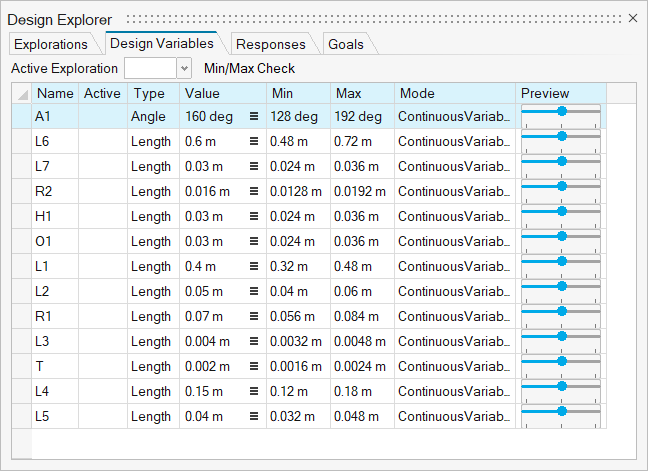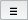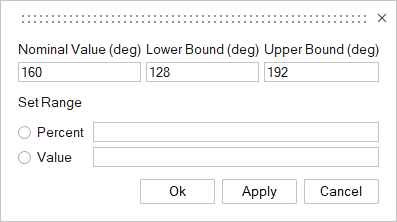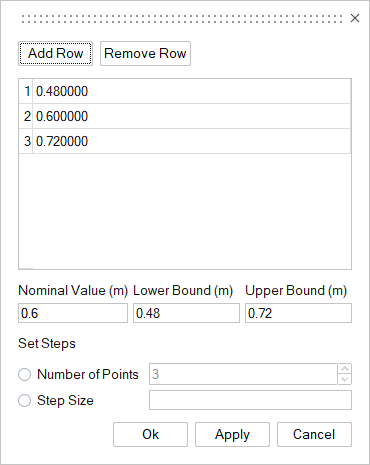# Design Variables

Design variables are changes of the geometric variables that influence the performance of certain analyses. Geometric variables may be sketch dimensions, lengths, angles, or push/pulls. Geometric constraints are minimum and maximum values for the defined variables. These constraints are specified in the Design Variable tab and the variable's value will vary within the exploration.

## Manage Design Variables

All variables in the Variable Manager are automatically added to the Design Variables manager, except if they are linked to other variables in the Variable Manager. In the Design Variables manager, you can set nominal values, the minimum and maximum values of the variable range to be studied, and the mode (continuous or discrete).

1. On the Design Explorer ribbon, select the Design Variables tool.You are now in the Design Variables step of the Design Explorer workflow.2. You can do the following:
To Do this
Open the Design Variable Bound Checker for all active variables Click the Min/Max Check button.

Then click the Check button in each row to ensure that the Min and Max combinations result in a valid model.

Rename a variable Select the relevant Name field and type the new name.
Activate/deactivate a variable In the Active column, click the corresponding field to turn on/off the variable.
Modify the value of a variable Select the relevant Value field and enter the new value. Or for more options, click.
• For a Continuous Variable, you can modify the following values:• Nominal Value: Default value of the variable; also serves as the initial value in an Optimization exploration.
• Lower Bound: The minimum value of the variable range to be studied.
• Upper Bound: The maximum value of the variable range to be studied.
• Set Range: Set the range between the minimum and maximum values based on a Percent or Value difference above and below the nominal value for a Continuous Variable. By default, the range is +/- 10% of the nominal value.
For a Discrete Variable, you can modify the following values:• Nominal Value: Default value of the variable; also serves as the initial value in an Optimization exploration.
• Lower Bound: The minimum value of the variable range to be studied.
• Upper Bound: The maximum value of the variable range to be studied.
• Set Steps: Set the number of steps between the minimum and maximum values based on the Number of Points or Step Size.
Define the minimum and maximum values of the variable range to be studied Select the relevant Min or Max field and enter the minimum or maximum value of the variable range to be studied.
Change the variable mode In the relevant Mode field, choose from a Continuous Variable or a Discrete Variable.
Preview variables Drag the Preview slider left and right to visualize changes.